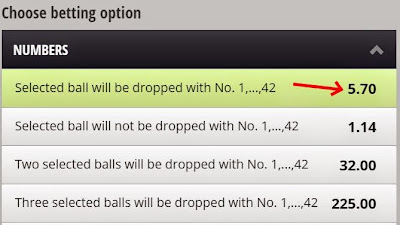### Definition of Betgames Africa Odds

Definition of Odds Display for Betgames Africa

Betgames Africa (BGA) Odds
The Betgames Africa odds are displayed in the European style. These odds include the player's stake.  This means that the total payout is worked our by multiplying the stake by the odds.What the odds look like on the Betgames Africa platform

So with the example off, the odds here are shown as 5.70.

This means that if you bet a R20 stake. your payout would be calculated as:

5.70 x R20 = R114

The payout you would be given is R114.  This includes your R20 stake.

To understand how it would be shown in South Africa, read below.

RSA Odds
These are the decimal fixed odds that are common to players in South Africa. The stake is not included in the odds.  Therefore these odds indicate what would be won if 1 unit was played, but does not include the stake. The stake will be returned along with the payout to the player.

If the European (BGA) odds above is 5.70, this includes the stake which is 1. Therefore the RSA odds would be displayed as 4.70 (5.70 - 1) as RSA odds does not include the stake. It still works out the same as the stake in RSA odds is returned to you in the payout.

4.70 x R20 = R94

R94 + R20 stake back = R114

Fractional Odds
These are the fractional fixed odds that are common to players in South Africa. This is the old way of calling odds, and is displayed here to communicate the equivalent to the BGA Odds. The decimal equivalent of fractional odds is calculated as a direct division of the fraction (left number divided by right number). Click here to view how fractional odds are equal to decimal odds.